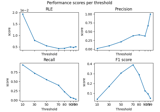# mne.simulation.metrics.precision_score#

mne.simulation.metrics.precision_score(stc_true, stc_est, threshold='90%', per_sample=True)[source]#

Compute the precision.

The precision is the ratio `tp / (tp + fp)` where `tp` is the number of true positives and `fp` the number of false positives. The precision is intuitively the ability of the classifier not to label as positive a sample that is negative.

The best value is 1 and the worst value is 0.

Threshold is used first for data binarization.

Parameters:
stc_trueinstance of (Vol|Mixed)SourceEstimate

The source estimates containing correct values.

stc_estinstance of (Vol|Mixed)SourceEstimate

The source estimates containing estimated values e.g. obtained with a source imaging method.

threshold

The threshold to apply to source estimates before computing the precision. If a string the threshold is a percentage and it should end with the percent character.

per_sample`bool`

If True the metric is computed for each sample separately. If False, the metric is spatio-temporal.

Returns:
metric`float` | `array`, shape (n_times,)

The metric. float if per_sample is False, else array with the values computed for each time point.

Notes

New in version 1.2.

## Examples using `mne.simulation.metrics.precision_score`#Compare simulated and estimated source activity

Compare simulated and estimated source activity